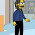## Friday, September 18, 2009

### And nows the time, the time is now

In my previous post I showed one way of breaking procedural abstraction. Dynamic binding allows the details of the implementation to be visible to unrelated code, and this causes unpredictable consequences (if it is used pervasively).

Let's give Louis Reasoner another tricky task. He's going to port the code to Scheme and he is to remove the logging from `lib-mapcar` and instead instrument the code with a counter that will be used by the statistics package. Recall that the code currently looks like this:
```(defun lib-mapcar (f list)
(cond ((consp list)
(let ((h (car list)))
(log "Calling " f " on " h)
(cons (funcall f h)
(lib-mapcar f (cdr list)))))
((null list) '())
(t (error "improper list"))))
```
The port to Scheme is easy:
```(define lib-mapcar
(lambda (f list)
(cond ((pair? list)
(let ((h (car list)))
(cons (f h)
(lib-mapcar f (cdr list)))))
((null? list) '())
(else (error "improper list")))))
```
And Louis adds a counter:
```(define lib-mapcar
(let ((calls-to-f 0))
(lambda (f list)
(cond ((pair? list)
(let ((h (car list)))
(cons (begin
(set! calls-to-f (+ calls-to-f 1))
(f h))
(lib-mapcar f (cdr list)))))
((null? list) '())
(else (error "improper list"))))))
```
But at this point he is stuck. He wants to be able to get at the value of the counter in order to read the count, but he can't because of the lexical scoping. There is an easy trick. We use another closure that closes over the same variable:
```(define *counters* '())

(set! *counters* (cons (cons name reader) *counters*)))

(define lib-mapcar
(let ((calls-to-f 0))
(add-counter! 'calls-to-f (lambda () calls-to-f))
(lambda (f list)
(cond ((pair? list)
(let ((h (car list)))
(cons (begin
(set! calls-to-f (+ calls-to-f 1))
(f h))
(lib-mapcar f (cdr list)))))
((null? list) '())
(else (error "improper list"))))))
```
And here it is in action:
```(lib-mapcar (lambda (x) (* x 2)) '(3 1 4 1 5 9 2 6 5 3 5))
=> (6 2 8 2 10 18 4 12 10 6 10)

((cdr (assq 'calls-to-f *counters*)))
=> 11
```
Using an alist to hold the counters and just invoking the reader procedure is a little crude (we could make some nice abstractions here), but that isn't the point. The point is that by closing over `calls-to-f` and exporting that closure we have poked a very tiny hole in our abstraction barrier. The hole is just big enough that some external code that is not under our control can read the value of our counter, but that is it. There is no way for the external code to modify the value. But there is one other thing we hid. The name of the variable that holds the counter is also hidden from the external code. If we want, we can change the code like this:
```(define lib-mapcar
(let ((the-counter 0))
(add-counter! 'calls-to-f (lambda () the-counter))
(lambda (f list)
(cond ((pair? list)
(let ((h (car list)))
(cons (begin
(set! the-counter (+ the-counter 1))
(f h))
(lib-mapcar f (cdr list)))))
((null? list) '())
(else (error "improper list"))))))
```
I have renamed the variable and all the places that the variable is used. This makes no difference to any other code. And because the scope is lexical, I know that all the code that could possibly care about the variable name is right there. I don't need to sift through the entire rest of the code base or obtain a list of variables from my customers. Nor do I have to tell them I changed the name. Now this is pretty cool.

You should find it easy to imagine how we could allow the external code to reset the counter to zero in addition to reading it, but not allow it to set the counter to an arbitrary value.

1.You should find it easy to imagine how we could allow the external code to reset the counter to zero in addition to reading it, but not allow it to set the counter to an arbitrary value.

And shortly thereafter, a poor man's object system was born... :-)

2.I did say it was crude.

I'm using lambda expressions to encapsulate and control access to state, which is one of the hallmarks of an object system. But I wouldn't suggest that you actually build an object system this way.